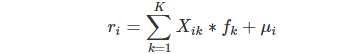# AI Risk Assessment Algorithm

AI's risk management advantages are more reflected in the financial service field. Artificial intelligence and big data are the right arms of financial risk control, and both are indispensable. The application in the evaluation of financial risks and returns can help solve the problem of information asymmetry, provide accurate user needs, and improve the efficiency of farming returns.
The high-quality knowledge graph provides an effective relationship between information, which is helpful to reduce the problem of information asymmetry; the deep learning method facilitates engine iteration, which is beneficial to provide users with the most suitable investment pool according to user needs. Based on a multi-factor model, the recommendation algorithm is conducive to predicting product risks and returns.
The expected return of the mining pool is the compensation for the risks undertaken by the profit farming participants, and the multi-factor model is a quantitative expression of the risk-return relationship. The multi-factor model quantitatively describes the linear relationship between the expected return rate of the mining pool and the factor load (risk exposure) of the mining pool on each factor, and the linear relationship between the factor return rate of each factor per unit factor load (risk exposure) . Multi-Factor Model (Multiple-Factor Model, MFM) is a complete risk model developed based on the idea of ​​the APT model.
The general expression of the multi-factor model:Where:
Xik = Factor exposure of pool i on factor k
fk = Factor return for k
μi = The residual rate of return of pool i